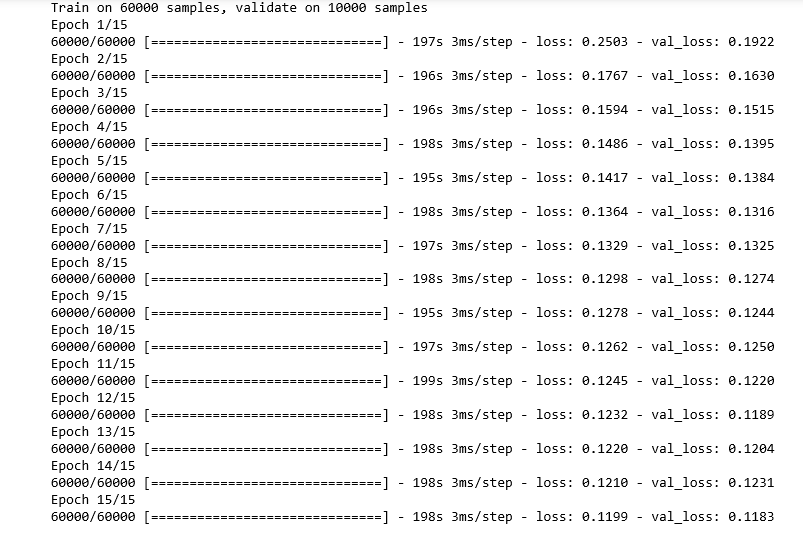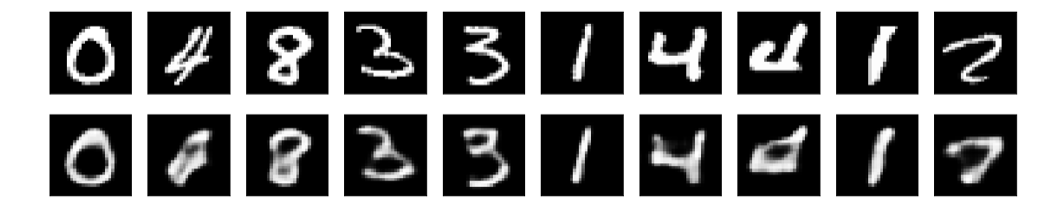# Building an Auto-Encoder using Keras

Prerequisites: Auto-encoders

This article will demonstrate the process of data compression and the reconstruction of the encoded data by using Machine Learning by first building an Auto-encoder using Keras and then reconstructing the encoded data and visualizing the reconstruction. We would be using the MNIST handwritten digits dataset which is preloaded into the Keras module about which you can read here.

The code is structured as follows: First all the utility functions are defined which are needed at different steps of the building of the Auto-encoder are defined and then each function is called accordingly.

Step 1: Importing the required libraries

 `import` `numpy as np ` `import` `matplotlib.pyplot as plt ` `from` `random ``import` `randint ` `from` `keras ``import` `backend as K ` `from` `keras.layers ``import` `Input``, Dense, Conv2D, MaxPooling2D, UpSampling2D ` `from` `keras.models ``import` `Model ` `from` `keras.datasets ``import` `mnist ` `from` `keras.callbacks ``import` `TensorBoard `

Step 2: Defining a utility function to load the data

 `def` `load_data(): ` `    ``# defining the input image size  ` `    ``input_image ``=` `Input``(shape ``=``(``28``, ``28``, ``1``)) ` `     `  `    ``# Loading the data and dividing the data into training and testing sets ` `    ``(X_train, _), (X_test, _) ``=` `mnist.load_data() ` `     `  `    ``# Cleaning and reshaping the data as required by the model ` `    ``X_train ``=` `X_train.astype(``'float32'``) ``/` `255.` `    ``X_train ``=` `np.reshape(X_train, (``len``(X_train), ``28``, ``28``, ``1``)) ` `    ``X_test ``=` `X_test.astype(``'float32'``) ``/` `255.` `    ``X_test ``=` `np.reshape(X_test, (``len``(X_test), ``28``, ``28``, ``1``)) ` `     `  `    ``return` `X_train, X_test, input_image `

Note: While loading the data, notice that the space where the training labels are loaded are kept empty because the compression process does not involve the output labels

Step 3: Defining a utility function to build the Auto-encoder neural network

 `def` `build_network(input_image): ` `     `  `    ``# Building the encoder of the Auto-encoder ` `    ``x ``=` `Conv2D(``16``, (``3``, ``3``), activation ``=``'relu'``, padding ``=``'same'``)(input_image) ` `    ``x ``=` `MaxPooling2D((``2``, ``2``), padding ``=``'same'``)(x) ` `    ``x ``=` `Conv2D(``8``, (``3``, ``3``), activation ``=``'relu'``, padding ``=``'same'``)(x) ` `    ``x ``=` `MaxPooling2D((``2``, ``2``), padding ``=``'same'``)(x) ` `    ``x ``=` `Conv2D(``8``, (``3``, ``3``), activation ``=``'relu'``, padding ``=``'same'``)(x) ` `    ``encoded_layer ``=` `MaxPooling2D((``2``, ``2``), padding ``=``'same'``)(x) ` `     `  `    ``# Building the decoder of the Auto-encoder ` `    ``x ``=` `Conv2D(``8``, (``3``, ``3``), activation ``=``'relu'``, padding ``=``'same'``)(encoded_layer) ` `    ``x ``=` `UpSampling2D((``2``, ``2``))(x) ` `    ``x ``=` `Conv2D(``8``, (``3``, ``3``), activation ``=``'relu'``, padding ``=``'same'``)(x) ` `    ``x ``=` `UpSampling2D((``2``, ``2``))(x) ` `    ``x ``=` `Conv2D(``16``, (``3``, ``3``), activation ``=``'relu'``)(x) ` `    ``x ``=` `UpSampling2D((``2``, ``2``))(x) ` `    ``decoded_layer ``=` `Conv2D(``1``, (``3``, ``3``), activation ``=``'sigmoid'``, padding ``=``'same'``)(x) ` `     `  `    ``return` `decoded_layer `

Step 4: Defining a utility function to build and train the Auto-encoder network

 `def` `build_auto_encoder_model(X_train, X_test, input_image, decoded_layer): ` `     `  `    ``# Defining the parameters of the Auto-encoder ` `    ``autoencoder ``=` `Model(input_image, decoded_layer) ` `    ``autoencoder.``compile``(optimizer ``=``'adadelta'``, loss ``=``'binary_crossentropy'``) ` `     `  `    ``# Training the Auto-encoder ` `    ``autoencoder.fit(X_train, X_train, ` `                ``epochs ``=` `15``, ` `                ``batch_size ``=` `256``, ` `                ``shuffle ``=` `True``, ` `                ``validation_data ``=``(X_test, X_test), ` `                ``callbacks ``=``[TensorBoard(log_dir ``=``'/tmp / autoencoder'``)]) ` `     `  `    ``return` `autoencoder `

Step 5: Defining a utility function to visualize the reconstruction

 `def` `visualize(model, X_test): ` `     `  `    ``# Reconstructing the encoded images ` `    ``reconstructed_images ``=` `model.predict(X_test) ` `     `  `    ``plt.figure(figsize ``=``(``20``, ``4``)) ` `    ``for` `i ``in` `range``(``1``, ``11``): ` `         `  `        ``# Generating a random to get random results ` `        ``rand_num ``=` `randint(``0``, ``10001``) ` `     `  `        ``# To display the original image ` `        ``ax ``=` `plt.subplot(``2``, ``10``, i) ` `        ``plt.imshow(X_test[rand_num].reshape(``28``, ``28``)) ` `        ``plt.gray() ` `        ``ax.get_xaxis().set_visible(``False``) ` `        ``ax.get_yaxis().set_visible(``False``) ` ` `  `        ``# To display the reconstructed image ` `        ``ax ``=` `plt.subplot(``2``, ``10``, i ``+` `10``) ` `        ``plt.imshow(reconstructed_images[rand_num].reshape(``28``, ``28``)) ` `        ``plt.gray() ` `        ``ax.get_xaxis().set_visible(``False``) ` `        ``ax.get_yaxis().set_visible(``False``) ` `         `  `    ``# Displaying the plot ` `    ``plt.show() `

Step 6: Calling the utility functions in the appropriate order

 `X_train, X_test, input_image ``=` `load_data() `

b) Building the network

 `decoded_layer ``=` `build_network(input_image) `

c) Building and training the Auto-encoder

 `auto_encoder_model ``=` `build_auto_encoder_model(X_train, ` `                                             ``X_test, ` `                                             ``input_image, ` `                                             ``decoded_layer) `d) Visualizing the reconstruction

 `visualize(auto_encoder_model, X_test) `My Personal Notes arrow_drop_upCheck out this Author's contributed articles.

If you like GeeksforGeeks and would like to contribute, you can also write an article using contribute.geeksforgeeks.org or mail your article to contribute@geeksforgeeks.org. See your article appearing on the GeeksforGeeks main page and help other Geeks.

Please Improve this article if you find anything incorrect by clicking on the "Improve Article" button below.

Article Tags :
Practice Tags :

Be the First to upvote.

Please write to us at contribute@geeksforgeeks.org to report any issue with the above content.